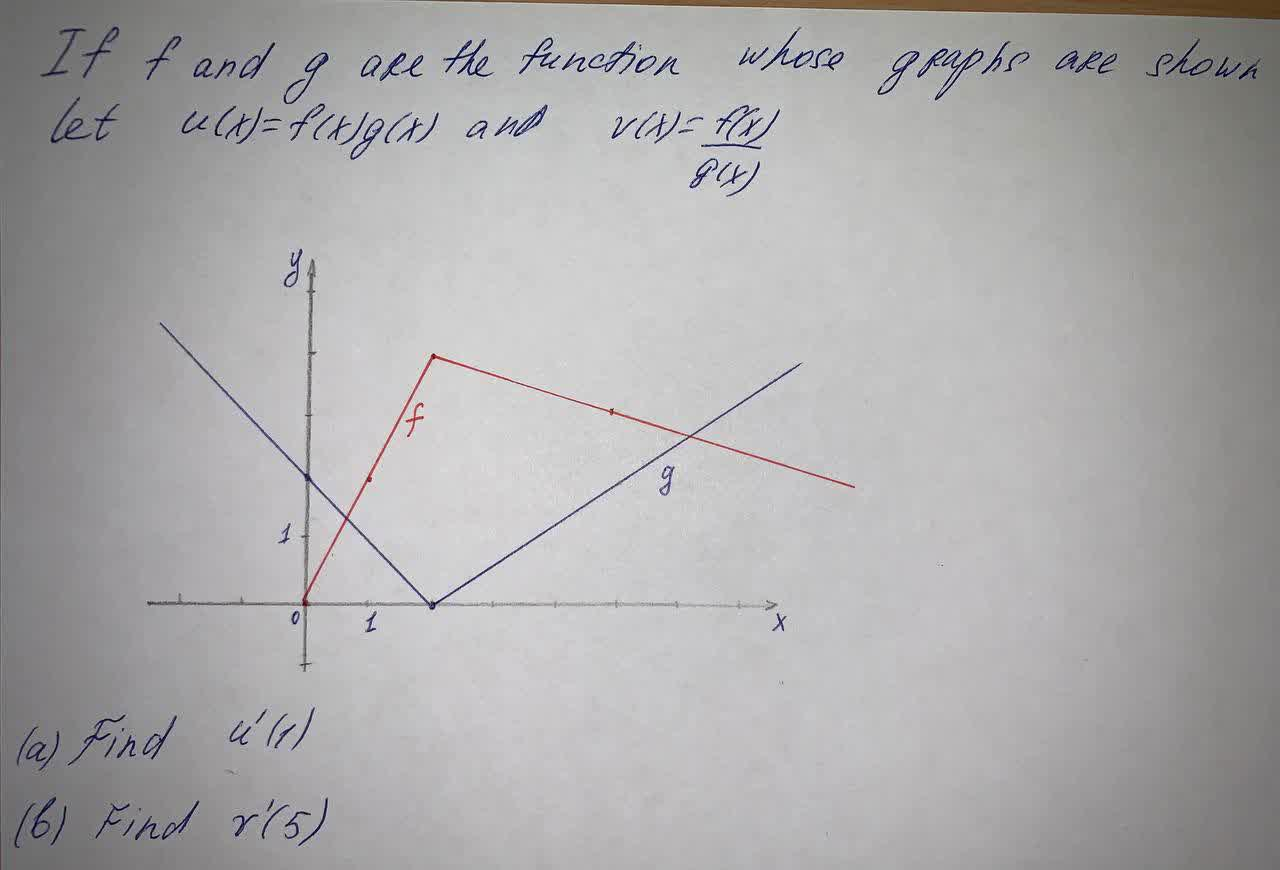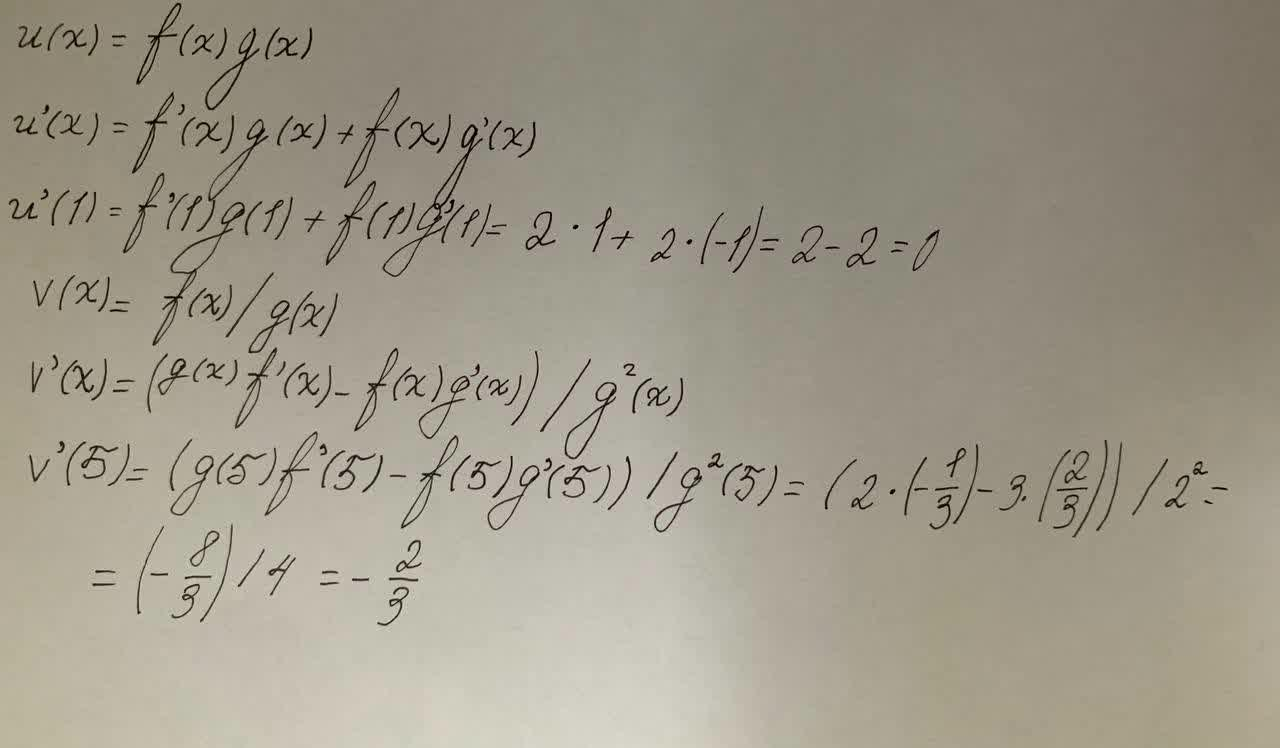Question# If f and g Are The Functions Whose Graphs Are Shown, Let u(x) = f(x)g(x) and v(x) = \frac{f(x)}{g(x)}

Parametric equations, polar coordinates, and vector-valued functions
ANSWEREDIf f and g Are The Functions Whose Graphs Are Shown, Let u(x) = f(x)g(x) and $$\displaystyle{v}{\left({x}\right)}={\frac{{{f{{\left({x}\right)}}}}}{{{g{{\left({x}\right)}}}}}}$$